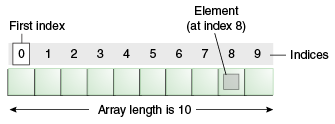Java™ 教程-Java Tutorials 中文版
Trail: Learning the Java Language
Lesson: Language Basics
Section: Variables

# 数组

array (数组) 是一个容器对象，它保存固定数量的单种类型的值。数组的长度是在创建数组时建立的。创建后，其长度是固定的。你已经在 "Hello World!" 应用的 `main` 方法中看到过一个数组的例子。本节更详细地讨论数组。10 个元素的数组。

```
class ArrayDemo {
public static void main(String[] args) {
// declares an array of integers
int[] anArray;

// allocates memory for 10 integers
anArray = new int;

// initialize first element
anArray = 100;
// initialize second element
anArray = 200;
// and so forth
anArray = 300;
anArray = 400;
anArray = 500;
anArray = 600;
anArray = 700;
anArray = 800;
anArray = 900;
anArray = 1000;

System.out.println("Element at index 0: "
+ anArray);
System.out.println("Element at index 1: "
+ anArray);
System.out.println("Element at index 2: "
+ anArray);
System.out.println("Element at index 3: "
+ anArray);
System.out.println("Element at index 4: "
+ anArray);
System.out.println("Element at index 5: "
+ anArray);
System.out.println("Element at index 6: "
+ anArray);
System.out.println("Element at index 7: "
+ anArray);
System.out.println("Element at index 8: "
+ anArray);
System.out.println("Element at index 9: "
+ anArray);
}
}
```

```Element at index 0: 100
Element at index 1: 200
Element at index 2: 300
Element at index 3: 400
Element at index 4: 500
Element at index 5: 600
Element at index 6: 700
Element at index 7: 800
Element at index 8: 900
Element at index 9: 1000
```

## 声明一个变量来引用一个数组

```// declares an array of integers
int[] anArray;
```

```byte[] anArrayOfBytes;
short[] anArrayOfShorts;
long[] anArrayOfLongs;
float[] anArrayOfFloats;
double[] anArrayOfDoubles;
boolean[] anArrayOfBooleans;
char[] anArrayOfChars;
String[] anArrayOfStrings;
```

```// this form is discouraged
float anArrayOfFloats[];
```

## 创建，初始化和访问数组

```// create an array of integers
anArray = new int;
```

```ArrayDemo.java:4: Variable anArray may not have been initialized.
```

```anArray = 100; // initialize first element
anArray = 200; // initialize second element
anArray = 300; // and so forth
```

```System.out.println("Element 1 at index 0: " + anArray);
System.out.println("Element 2 at index 1: " + anArray);
System.out.println("Element 3 at index 2: " + anArray);
```

```int[] anArray = {
100, 200, 300,
400, 500, 600,
700, 800, 900, 1000
};
```

```class MultiDimArrayDemo {
public static void main(String[] args) {
String[][] names = {
{"Mr. ", "Mrs. ", "Ms. "},
{"Smith", "Jones"}
};
// Mr. Smith
System.out.println(names + names);
// Ms. Jones
System.out.println(names + names);
}
}
```

```Mr. Smith
Ms. Jones
```

``` System.out.println(anArray.length);
```

## 复制数组

`System` 类有一个 `arraycopy` 方法，你可以使用该方法将数据从一个数组有效地复制到另一个数组中：

```public static void arraycopy(Object src, int srcPos,
Object dest, int destPos, int length)
```

```
class ArrayCopyDemo {
public static void main(String[] args) {
char[] copyFrom = { 'd', 'e', 'c', 'a', 'f', 'f', 'e',
'i', 'n', 'a', 't', 'e', 'd' };
char[] copyTo = new char;

System.arraycopy(copyFrom, 2, copyTo, 0, 7);
System.out.println(new String(copyTo));
}
}
```

```caffein
```

## 数组操作

```
class ArrayCopyOfDemo {
public static void main(String[] args) {

char[] copyFrom = {'d', 'e', 'c', 'a', 'f', 'f', 'e',
'i', 'n', 'a', 't', 'e', 'd'};

char[] copyTo = java.util.Arrays.copyOfRange(copyFrom, 2, 9);

System.out.println(new String(copyTo));
}
}
```

`java.util.Arrays` 类中的方法提供的一些其他有用的操作有：

• 在数组中搜索特定值以获取它所在的索引(`binarySearch` 方法)。
• 比较两个数组以确定它们是否相等(`equals` 方法)。
• 填充数组以在每个索引处放置特定值(`fill` 方法)。
• 按升序排列数组。这可以使用 `sort` 方法顺序排序，或使用 Java SE 8 中引入的 `parallelSort` 方法并行排序。多处理器系统上的大型数组的并行排序比顺序数组排序要快。

Previous page: Primitive Data Types
Next page: Summary of Variables